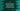# C program to get the integer and fraction or decimal part## C program to get the integer and fraction or decimal part:

In this post, we will learn how to get the integer and fraction or decimal part from a given floating-point number in C. Our program will take the floating-point number as an input from the user and it will print the integer part and the fraction part.

We can solve this problem in different ways in C. Let’s have a look at them one by one.

## Method 1: By casting the number to integer:

In this method, we can cast the number to an integer by using (int) typecasting. Once we get the integer part, we can easily calculate the decimal part by finding the difference between the given number and integer part.

Below is the complete program:

``````#include <stdio.h>

int main()
{
float num;

printf("Enter a number: ");
scanf("%f", &num);

int num_integer = (int)num;
float num_decimal = num - num_integer;

printf("Integer part: %d, Decimal part: %f\n", num_integer, num_decimal);

return 0;
}``````

In this program,

• num is a floating-point variable to store the user input number.
• It asks the user to enter a number, read it and stores it in num.
• num_integer is the integer part of the number num. We are casting it to an integer to get the integer part.
• num_decimal is the decimal or fraction part. We are subtracting the value of num_integer from num to get this value.
• The last printf statement is printing both integer and decimal part.

If you run this program, it will print output as like below:

``````Enter a number: 12.3456
Integer part: 12, Decimal part: 0.345600

Enter a number: 99.99
Integer part: 99, Decimal part: 0.989998

Enter a number: 12.45
Integer part: 12, Decimal part: 0.450000``````

## Method 2: By using modf function:

modf is an inbuilt function defined in math.h header file. We can use this function to convert a floating point variable to integer and fractional or decimal part.

This method is defined as like below:

``double modf(double v, double *p)``
• v is the floating-point value we want to divide into fractional and decimal parts.
• p is a pointer to store the integer part.

It returns the fractional part of v.

### Example C program:

The below program uses modf to get the integer and fractional part from a given number.

``````#include <stdio.h>
#include <math.h>

int main()
{
double num, num_integer, num_decimal;

printf("Enter a number: ");
scanf("%lf", &num);

num_decimal = modf(num, &num_integer);

printf("Integer part: %lf, Decimal part: %lf\n", num_integer, num_decimal);

return 0;
}``````

Here,

• num, num_integer and num_decimal are three double variables to hold the user input number, the integer part and decimal part of the number.
• It asks the user to enter a number and reads it in the num variable.
• By using modf, it calculates the integer and decimal part.
• The last printf is printing the integer part,and decimal part of the number.

If you run this program, it will print output as like below:

``````Enter a number: 12.3456
Integer part: 12.000000, Decimal part: 0.345600

Enter a number: 99.99
Integer part: 99.000000, Decimal part: 0.990000

Enter a number: 12.45
Integer part: 12.000000, Decimal part: 0.450000``````